https://doi.org/10.5194/ejm-32-27-2020
https://doi.org/10.5194/ejm-32-27-2020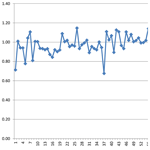# Determination of the H2O content in minerals, especially zeolites, from their refractive indices based on mean electronic polarizabilities of cations

Reinhard X. Fischer, Manfred Burianek, and Robert D. Shannon
Abstract

It is shown here that the H2O content of hydrous minerals can be determined from their mean refractive indices with high accuracy. This is especially important when only small single crystals are available. Such small crystals are generally not suitable for thermal analyses or for other reliable methods of measuring the amount of H2O. In order to determine the contribution of the H2O molecules to the optical properties, the total electronic polarizability is calculated from the anhydrous part of the chemical composition using the additivity rule for individual electronic polarizabilities of cations and anions. This anhydrous contribution is then compared with the total observed electronic polarizability calculated from the mean refractive index of the hydrous compound using the Anderson–Eggleton relationship. The difference between the two values represents the contribution of H2O. The amount can be derived by solving the equation ${\mathit{\alpha }}_{\mathrm{calc}}=\sum _{i}{n}_{i}{\mathit{\alpha }}_{i\mathrm{cat}}+\sum _{j}\left({\mathit{\alpha }}_{j}^{o}×{\mathrm{10}}^{-\left[\frac{{N}_{j}}{{V}_{\mathrm{m}}^{\mathrm{1.2}}}×{\left({n}_{j}+{n}_{W}\right)}^{\mathrm{1.2}}\right]}\right)+{n}_{w}×{\mathit{\alpha }}_{W}$ for the number nw of H2O molecules per formula unit (pfu), with the electronic polarizabilities αcat for cations, the values N and αo describing the anion polarizabilities, the number n of cations and anions, and the molar volume Vm, using a value of αW=1.62 Å3 for the electronic polarizability of H2O. The equation is solved numerically, yielding the number nw of H2O molecules per formula unit. The results are compared with the observed H2O content evaluating 157 zeolite-type compounds and 770 non-zeolitic hydrous compounds, showing good agreement. This agreement is expressed by a factor relating the calculated to the observed numbers being close to 1 for the majority of compounds. Zeolites with occluded anionic or neutral species (SO3, SO4, CO2, or CO3) show unusually high deviations between the calculated and observed amount of H2O, indicating that the polarizabilities of these species should be treated differently in zeolites and zeolite-type compounds.

Share
Dates
1 Introduction

The water content of hydrous minerals and synthetic compounds is usually determined by thermogravimetric methods recording the weight loss upon dehydration at an increasing temperature. If the chemical composition of the anhydrous part of the compound is known, its formula weight can be calculated along with the number of H2O molecules representing the weight loss. Whereas the cation content can be derived from microchemical analyses, e.g., by electron microprobe analyses (EMPA) or analytical transmission electron microscopy (ATEM), on species with a size of a few microns, thermoanalytical methods for the determination of the water content require an amount of a sample in the range of a few milligrams. The same applies to carbon hydrogen and nitrogen (CHN) analyzers which usually need a few milligrams for accurate analyses. Alternatively, the H2O content could be derived from the measured density if the specimen is big enough for the experimental determination of the density. The determination of the water content becomes less accurate if lower amounts or even just one single crystal with a size of about 100 µm and a mass of a few micrograms or less exists.

It is well known that the optical properties vary with the amount of H2O in a crystalline compound. We mention two examples: Hey and Bannister (1932a) studied the effect of dehydration on the optical properties of natrolite, and Medenbach et al. (1980) investigated the variation in the refractive index of synthetic Mg-cordierite with H2O content, finding a linear relationship between the mean refractive index and the weight fraction of H2O. All studies we know so far are empirical descriptions of the dependence of the refractive indices on the H2O content derived by calibration curves for a special system of compounds. Here, we describe a theoretical approach for determining the H2O content from mean refractive indices of compounds with known chemical composition of the anhydrous part. We show that the number of H2O molecules per formula unit (pfu) can be determined with high accuracy from the mean refractive indices of hydrous crystals.

A special focus is on the zeolite group of minerals, representing a large and popular group of hydrous minerals. Zeolites represent one of the most important classes of materials, used as catalysts in oil refineries for the production of gasoline and as ion exchangers as an additive, e.g., in household detergents for the softening of water. Following the definition of the subcommittee on zeolites of the International Mineralogical Association (Coombs et al., 1998), “A zeolite mineral is a crystalline substance with a structure characterized by a framework of linked tetrahedra, each consisting of four O atoms surrounding a cation. This framework contains open cavities in the form of channels and cages. These are usually occupied by H2O molecules and extra-framework cations that are commonly exchangeable”.

Table 1Entries of zeolite-type compounds used for the comparison of observed and calculated H2O content.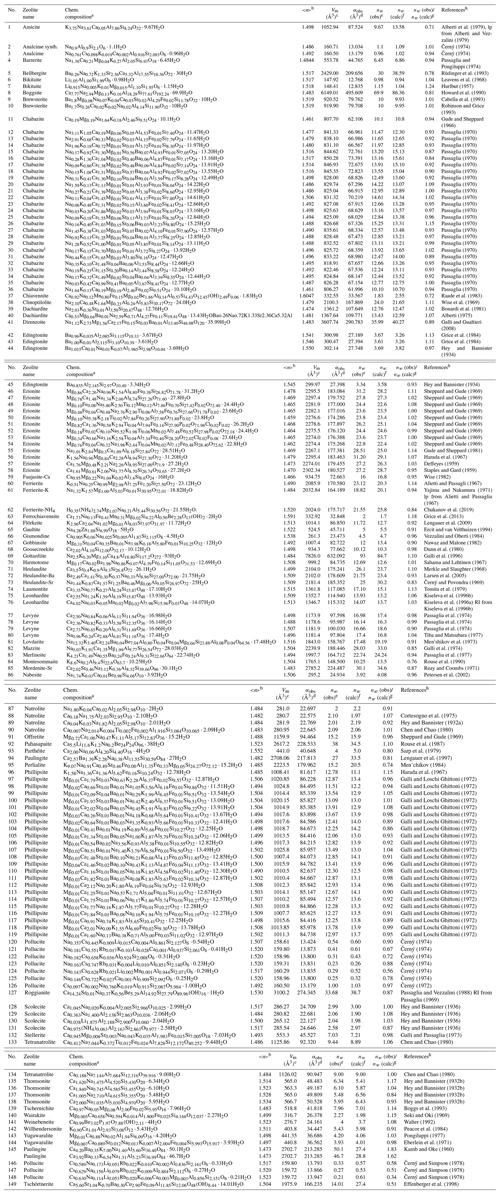a Chemical composition normalized according to the formula unit as listed in Coombs et al. (1998). b Mean refractive index $<\phantom{\rule{-0.125em}{0ex}}\phantom{\rule{-0.125em}{0ex}}n\phantom{\rule{-0.125em}{0ex}}\phantom{\rule{-0.125em}{0ex}}>$. c Molar volume Vm per formula unit. d Observed total electronic polarizability calculated
from mean refractive index using Eq. (1). e Observed number of H2O molecules per formula unit as given in the respective literature. f Number of H2O molecules per formula calculated as explained in the text, using the
program POLARIO (Fischer et al., 2018). g Factor relating the calculated to the observed H2O content. h lp is lattice parameters, and RI is refractive index. i Chabazite with composition
Na0.08K0.42Ca0.84Mg0.29Sr0.03Ba0.09Al3.17Fe0.08Si8.81O24⋅10.84H2O was omitted because of Fe2O3 impurities (Passaglia, 1970).

2 Theoretical background

Our approach is based on the fact that the total electronic polarizability of a mineral or synthetic compound can be calculated from the sum of the individual contributions of the electronic polarizabilities αcat of cations and αan of the anions, following the procedure described by Shannon and Fischer (2016). Thus, the total polarizability of the anhydrous compound can be calculated from the chemical composition which then can be compared with the total polarizability derived from the observed mean refractive indices of the hydrous compound. The difference represents the contribution of the H2O molecules. To obtain the H2O content, refractive indices are measured, e.g., by immersion methods with a spindle stage on a petrographic microscope. Anisotropic indices are averaged according to $\left({n}_{x}+{n}_{y}+{n}_{z}\right)/\mathrm{3}$ or $\left(\mathrm{2}{n}_{o}+{n}_{e}\right)/\mathrm{3}$, yielding the mean refractive index $<\phantom{\rule{-0.125em}{0ex}}\phantom{\rule{-0.125em}{0ex}}n\phantom{\rule{-0.125em}{0ex}}\phantom{\rule{-0.125em}{0ex}}>$.

The total observed polarizability of the hydrous compound is then calculated from the mean refractive index $<\phantom{\rule{-0.125em}{0ex}}\phantom{\rule{-0.125em}{0ex}}n\phantom{\rule{-0.125em}{0ex}}\phantom{\rule{-0.125em}{0ex}}>$ using the Anderson–Eggleton relationship (Anderson, 1975; Eggleton, 1991; see Shannon and Fischer, 2016, for an explanation):

$\begin{array}{}\text{(1)}& {\mathit{\alpha }}_{\mathrm{obs}}=\frac{\left({n}^{\mathrm{2}}-\mathrm{1}\right){V}_{\mathrm{m}}}{\mathrm{4}\mathit{\pi }+\left(\frac{\mathrm{4}\mathit{\pi }}{\mathrm{3}}-\mathrm{2.26}\right)\left({n}^{\mathrm{2}}-\mathrm{1}\right)},\end{array}$

with the molar volume Vm of one formula unit.

The total electronic polarizability of the anhydrous compound can be calculated from the sum of the individual ion contributions (Shannon and Fischer, 2016) according to

$\begin{array}{}\text{(2)}& {\mathit{\alpha }}_{\mathrm{calc}}=\sum _{i=\mathrm{1}}^{{N}_{\mathrm{cat}}}{m}_{i}×{\mathit{\alpha }}_{i\mathrm{cat}}+\sum _{i=\mathrm{1}}^{{N}_{\mathrm{an}}}{n}_{i}×{\mathit{\alpha }}_{\mathrm{ian}},\end{array}$

with

$\begin{array}{}\text{(3)}& {\mathit{\alpha }}_{\mathrm{an}}={\mathit{\alpha }}_{-}^{o}×{\mathrm{10}}^{-{N}_{o}/{V}_{\mathrm{an}}^{\mathrm{1.2}}},\end{array}$

where αcat is taken from Table 4 and ${\mathit{\alpha }}_{-}^{o}$ and No from Table 5 in Shannon and Fischer (2016), and the anion volume Van is calculated from the molar volume Vm divided by the number of anions and H2O molecules.

The difference between observed and calculated polarizabilities $\mathrm{\Delta }=\left({\mathit{\alpha }}_{\mathrm{obs}}-{\mathit{\alpha }}_{\mathrm{calc}}\right)$ is due to the contribution of H2O. However, Δ cannot be simply divided by the electronic polarizability 1.62 Å3 of H2O because it is treated like an anion and not like a cation (Shannon and Fischer, 2016). Therefore, the number nw of H2O molecules has an influence on the calculation of the anion volume Van in Eq. (3), so it is needed to calculate the contribution of the anions even for the anhydrous part of the compound.

If we combine Eqs. (2) and (3) and separate the contribution of H2O from the other anions, we get

$\begin{array}{}\text{(4)}& \begin{array}{rl}{\mathit{\alpha }}_{\mathrm{calc}}=& \sum _{i}{n}_{i}{\mathit{\alpha }}_{i\mathrm{cat}}+\sum _{j}\left({\mathit{\alpha }}_{j}^{o}×{\mathrm{10}}^{-\left[\frac{{N}_{j}}{V}×{\left({n}_{j}+{n}_{W}\right)}^{\mathrm{1.2}}\right]}\right)\\ & +{n}_{w}×{\mathit{\alpha }}_{W},\end{array}\end{array}$

with $V={V}_{\mathrm{m}}^{\mathrm{1.2}}$, the number nw of H2O molecules per formula unit, and the electronic polarizability αW=1.62 Å3 of H2O.

Replacing αcalc in Eq. (4) by αobs from Eq. (1) and solving for nw yields the number of H2O molecules per formula unit.

The solution is done numerically using the program POLARIO (Fischer et al., 2018).

3 Dataset

We tested the approach on hydrous minerals with known chemical composition, water content, and refractive indices, taken from Table S1 in Shannon et al. (2017) and additional entries compiled here. The group of zeolite minerals is treated separately because it represents one of the most important classes of hydrous minerals and because we used mean electronic polarizability for the calculation of their total polarizabilities in contrast to the non-zeolitic minerals, where the total polarizabilities are calculated from electronic polarizabilities of cations with specific coordination numbers (CNs). Whereas the cation coordination is known for most of the non-zeolitic minerals, it is not clearly determined for most of the zeolite species where the chemical composition and the refractive indices have been determined but not the crystal structure. Therefore, Table 1 lists the results on 149 zeolites obtained with mean electronic polarizabilities, and Table S1 in the Supplement lists the results on 770 non-zeolitic minerals and synthetic compounds based on electronic polarizabilities taken from Table 4 in Shannon and Fischer (2016) for specific coordination numbers of cations. For the zeolites, the mean electronic polarizabilities of a cation in a certain oxidation state is calculated as the average of all cations of the same kind with different coordination numbers weighted by the number of entries used to determine the polarizability (see Table 4 in Shannon and Fischer, 2016). The mean values are listed in Table 2. They are internally stored in POLARIO (Fischer et al., 2018) used to calculate the water content of zeolites in Table 1. Zeolite entries are selected if they belong to one of the 237 types listed in the Database of Zeolite Structures of the International Zeolite Association (Baerlocher and McCusker, 2019). Thus, it also contains minerals like cancrinite which are not considered to be zeolite minerals but topologically have a zeolite-type framework. Only those entries are included in Table 1 where the optical data, the chemical composition, and the unit-cell parameters are available for the same specimen. There are a few exceptions where it was clear that the data from different publications can be assigned to the same crystal. We were aiming for a dataset large enough to have a representative selection and not necessarily for completeness.

All entries in Table 1 conform to the criteria listed above. However, the entries at the end of Table 1 (no. 145 to 149) show unusual deviations between observed and calculated H2O content which are assumed to be due to uncertainties in the chemical composition. Zeolites and zeolite-type compounds having occluded anionic or neutral species also show high deviations and are listed separately in Table 3. Details are discussed below. Not considered in this compilation of hydrous minerals are compounds containing elements where the electronic polarizabilities have not been determined in Shannon and Fischer (2016). These are, for example, cations with lone-pair electrons (Tl+, Sn2+, Pb2+, As3+, Sb3+, Bi3+, S4+, Se4+, Te4+, Cl5+, Br5+, and I5+) which do not fit the simple scheme of additivity. Also excluded from the compilation are compounds with sterically strained structures (Gagné et al., 2018) and some compounds with corner-shared or edge-shared octahedral networks (see Shannon and Fischer, 2016; Shannon et al., 2017) showing systematic deviations between observed and calculated polarizabilities.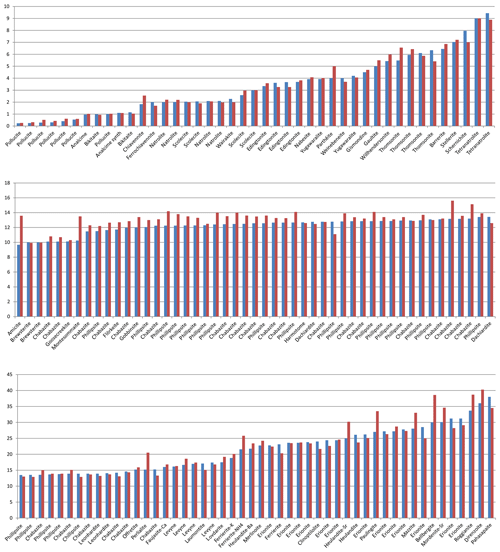Figure 1Histogram of observed (blue) and calculated (red) water content. Entries 145 to 149 are omitted (see text for explanation). The two entries with H2O content >50 (8 – boggsite; 69 – gottardiite) are not shown.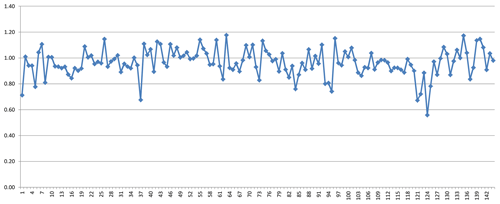Figure 2Plot of factors relating calculated to observed amount of H2O in zeolite-type minerals. The sequence from left to right follows the sequence of entries in Table 1. Entries 145 to 149 are omitted (see text for explanation).

4 Example

The approach used here is demonstrated in the example of synthetic analcime (entry 2 in Table 1) having the chemical composition Na0.9Al0.9Si2.1O6 ⋅1.1H2O, with data from sample 1 in Table 1 of Černý (1974). The following steps are taken to determine the water content from the refractive indices.

1. The observed total electronic polarizability αobs is calculated using Eq. (1) with Vm=160.71 Å3 (unit-cell volume from Table 1 in Černý, 1974, V=2571.35 Å3, divided by the number of formula units, Z=16) and isotropic n=1.486, yielding αobs=13.034 Å3.

2. The calculated total electronic polarizability αcalc of the anhydrous part of the chemical composition is calculated using the additivity rule with the individual electronic polarizabilities of ions from Shannon and Fischer (2016). Because the coordination number (CN) of Na is not determined in analcime (Černý, 1974), a mean value for Na with different CNs is used calculated according to α(Na) $=\left(\mathrm{17}\cdot \mathrm{0.760}+\mathrm{27}\cdot \mathrm{0.650}+\mathrm{207}\cdot \mathrm{0.560}+\mathrm{97}\cdot \mathrm{0.490}+\mathrm{197}\cdot \mathrm{0.430}+\mathrm{49}\cdot \mathrm{0.380}+\mathrm{25}\cdot \mathrm{0.340}+\mathrm{5}\cdot \mathrm{0.300}+\mathrm{9}\cdot \mathrm{0.270}\right)/\mathrm{633}=\mathrm{0.489}$ Å3, with values taken from Table 4 in Shannon and Fischer (2016). For the framework atoms Al and Si, the respective values for CN =4 are used. Electronic polarizabilities and the mean values are internally stored in the program POLARIO (Fischer et al., 2018), which is used for all calculations. Thus, αcalc(anhydrous) $=\mathrm{0.9}\cdot \mathit{\alpha }$(Na) $+\mathrm{0.9}\cdot \mathit{\alpha }$(Al) $+\mathrm{2.1}\cdot \mathit{\alpha }$(Si) $+\mathrm{6}\cdot \mathit{\alpha }$(O) $=\mathrm{0.9}\cdot \mathrm{0.489}+\mathrm{0.9}\cdot \mathrm{0.533}+\mathrm{2.1}\cdot \mathrm{0.284}+\mathrm{6}\cdot \mathrm{1.688}=\mathrm{11.439}$ Å3, where α(O) is calculated using Eq. (3), with ${\mathit{\alpha }}_{-}^{o}=\mathrm{1.79}$ Å3 and N=1.776 Å3 taken from Table 5 in Shannon and Fischer (2016) and ${V}_{\mathrm{an}}={V}_{\mathrm{m}}/\mathrm{6}=\mathrm{26.79}$ Å3.

3. The difference ${\mathit{\alpha }}_{\mathrm{obs}}-{\mathit{\alpha }}_{\mathrm{calc}}=\mathrm{1.595}$ Å3 represents the contribution of H2O to the total electronic polarizability. The individual electronic polarizability of H2O taken from Table 5 in Shannon and Fischer (2016) is α( H2O) =1.62 Å3. However, the simple determination of the number of H2O molecules nw pfu according to ${n}_{w}=\mathrm{1.595}/\mathrm{1.62}=\mathrm{0.98}$ is not correct because nw adds to the anion volume (Van=V(O) +V(H2O) $={V}_{\mathrm{m}}/\left({n}_{\mathrm{O}}+{n}_{w}\right)$ and thus contributes to the polarizability of the anhydrous part as well as that expressed in Eq. (4). Only the solution of Eq. (4) solved for nw yields the correct value. This is done numerically in POLARIO, yielding 1.09 H2O molecules pfu, which compares well with the observed 1.100 molecules determined in Černý (1974).

Alternatively, the H2O content could be calculated using the Gladstone–Dale approach after Mandarino (1976), where the refractive index n is calculated from $n={K}_{c}\cdot D+\mathrm{1}$ with ${K}_{c}=\sum _{i}\frac{{k}_{i}{p}_{i}}{\mathrm{100}}$ and where ki values are Gladstone–Dale constants, pi values are weight percentages, and D is the density (see also Shannon and Fischer, 2016). For the example of analcime, the resulting number of H2O molecules would be 0.89 H2O per formula unit using Gladstone–Dale constants from Mandarino (1981) and Eggleton (1991). A detailed comparison between our polarizability approach and the Gladstone–Dale concept will be published in a follow-up paper.

5 Comparison between observed and calculated H2O content in hydrous minerals

As mentioned above, the group of zeolite minerals was studied separately using mean electronic polarizabilities for the calculations. Figure 1 shows the water content calculated with Eq. (4) compared with the water content derived from analytical determinations as published in the respective references listed in Table 1. The factors relating the calculated to the observed values are shown in Fig. 2. It clearly demonstrates that the calculated values show a reasonable fit with the observed amount of H2O, with factors f=nw(obs)nw(calc) being close to 1. Outliers in Figs. 1 and 2 are mainly explained by uncertainties in the determination of chemical compositions and/or refractive indices.

Table 2Electronic polarizabilities α of cations from Table 4 in Shannon and Fischer (2016) averaged over different coordination numbers, including NH4 and five HxOy species.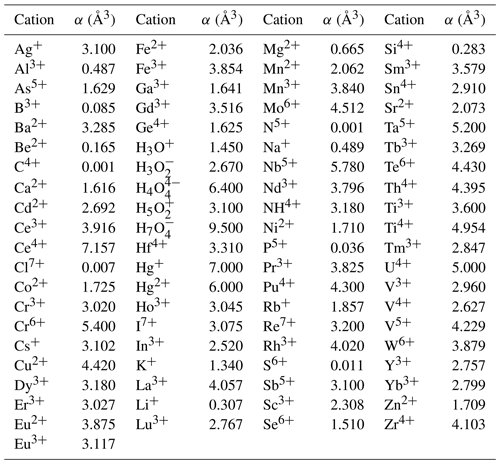In paulingite (145), something must be wrong in the chemical composition. The authors state the following: “The Al∕Si ratio of 0.15 given by the analysis conflicts with the cation composition … which requires an Al∕Si ratio of 0.47. Either the analysis is internally inconsistent, or else anions must be present outside the tectosilicate framework” (Kamb and Oke, 1960). The composition given in Table 1 is scaled to 42 framework cations and fixed to 84 O atoms corresponding to a factor of 3.5 relative to the original composition given in Kamb and Oke (1960), whereas 89.95 O atoms would be needed for charge compensation, which, however, does not represent a TO2 framework. Alternatively, in the second line 145 in Table 1 the non-oxygen content is scaled down to achieve charge balance. Then the factor relating calculated and observed H2O content changes from 1.83 to 1.62, which is lower but still rather high. In later work, Lengauer et al. (1997) investigated paulingite (94) with a different composition with 27 H2O molecules derived from thermogravimetric analysis (TGA) and 33.5 H2O calculated from the refractive index, yielding a factor of 0.81. The misfits for pollucite (146–148) might be due to errors in the chemical composition and/or the determination of the H2O content. According to Beger (1969) the sum of Cs + H2O should be equal to 1, whereas it is 0.34, for example, in pollucite (148). In tschörtnerite (149), “A quantitative determination of H2O∕OH was not possible because of the small amount of material available” (Effenberger et al., 1998). A number of 14 molecules pfu was calculated by difference from electron microprobe analyses (EMPAs), and 20 H2O were determined from the crystal-structure analysis. A loss of H2O is assumed due to the high-vacuum measuring conditions in the EMPA: “A part of the total amount of H2O is given as OH in order to achieve charge balance” (Effenberger et al., 1998). In total, this yields high uncertainties concerning the real H2O content in tschörtnerite. The authors assume an H2O content ≥20, which is much closer to the amount of 27.4 molecules calculated by us.

There are nine compounds listed in Table 3 containing anionic and/or neutral species (SO3, SO4, CO2, or CO3) occluded in the zeolite cavities. All these compounds show unusually high deviations between the observed and calculated amount of H2O. It is apparent that these species in zeolites must be treated separately in our concept, with individual electronic polarizabilities deviating from other sulfates, sulfites, and carbonates. For liottite (156 in Table 3; Merlino and Orlandi, 1977b) the number of H2O molecules was even calculated to be negative because the total polarizability of the anhydrous part of the compound was already higher than the corresponding observed value. However, it was shown in subsequent work by Ballirano et al. (1996) that it does not contain H2O, which might confirm our findings in addition to the problem with the anionic species.

Table 3Entries of zeolite-type compounds with occluded anionic species. Numbering continues to the sequence in Table 1.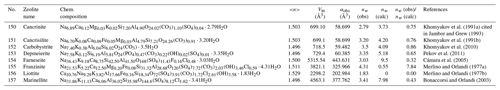The mean value of the factor relating the calculated amount of H2O to the observed one is more or less meaningless because high and low factors, each representing large errors, level each other out. More significant is the distribution of factors as shown in Fig. 3, where the bars in the histogram represent the frequency of occurrence of factors within a range of factors indicated on the horizontal axis. The mode is between 0.9 and 1.0, with 64 % of the factors between 0.9 and 1.1 and 86 % between 0.8 and 1.2.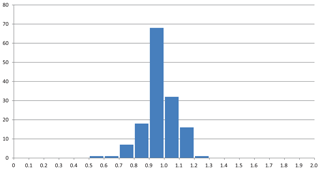Figure 3Frequency of occurrence of the factors for the zeolite entries 1–144 listed in Table 1 and shown in Fig. 2. (a) Factors calculated from polarizability analysis using different electronic polarizabilities for cations with different coordination numbers (from Table 4 in Shannon and Fischer, 2016). (b) Factors calculated from polarizability analysis using mean electronic polarizabilities from Table 2.

The situation is similar for the non-zeolitic minerals listed in Table S1, with 770 entries. The factors relating the calculated number of H2O molecules to the observed ones are plotted in Fig. 4, which shows a few outliers caused by inaccuracies in the chemical compositions, difficulties in the measurement of the refractive indices, or the observed H2O content just estimated or calculated and not determined by thermal analyses. Lotharmeyerite (entry 675) shows the largest deviation from 1 in Fig. 4a and b because of uncertainties in the observed OH (partially disordered) and H2O content (Yang et al., 2012). Because the majority of the entries show a reasonable fit with factors close to 1, we do not discuss here specific reasons for deviations. It should be noted that these factors are calculated irrespective of the precision of the number of molecules listed in the original publications. The number of H2O molecules for the first 30 entries in Table S1, for example, is given in integral numbers by the authors but calculated with all decimal places. If the calculated values are rounded to integers, they correspond exactly to the observed numbers except chukhrovite (27), where 12 H2O molecules are measured and 11.28 are calculated.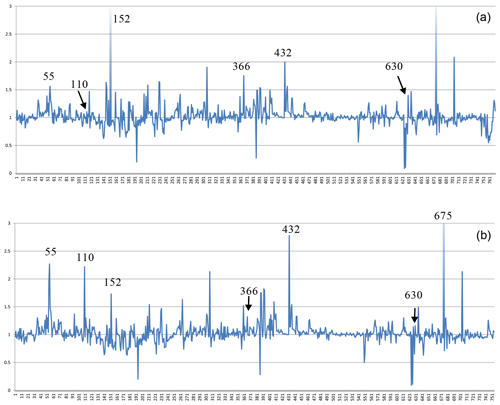Figure 4Plot of factors relating calculated to observed amount of H2O in non-zeolitic minerals. The sequence from left to right follows the sequence of entries in Table S1.

Thus, there are various sources of possible deviations. This might include rounded numbers, especially for low H2O content, where a number of 1 H2O molecule could be anything between 0.5 and 1.5 and therefore might already represent an error of 50 %. Other sources of errors are uncertainties in the chemical compositions, especially when they are based on energy-dispersive X-ray spectroscopy (EDX) analyses, estimated and not experimentally determined H2O content, insufficient quality of crystals for accurate measurements of the refractive indices, and also errors in our empirically determined electronic polarizabilities of cations and anions (Shannon and Fischer, 2016), which are derived in least-squares procedures. The cumulation of such errors will be reflected by factors significantly deviating from 1. Despite these influences, the data shown in Figs. 1 to 5 clearly show that there is on average a very good fit between the observed and calculated amount of H2O. In general the best results are achieved using the individual electronic polarizabilities of cations with specific coordination numbers (Table 4 in Shannon and Fischer, 2016). However, mean polarizabilities (Table 2) yield results that are sufficiently accurate if the CN of cations has not been determined. This is shown in Fig. 4, comparing the two approaches yielding essentially similar results with a few outliers. Entries 55 (priceite), 110 (calcioancylite-Nd), and 432 (bassanite), for example, have factors much higher for mean polarizabilities, but entries 152 (milarite), 366 (Li2SO4 H2O), and 630 (martyite), for example, have factors closer to 1 as compared with the corresponding values from the individual polarizabilities. Generally we recommend verifying questionable results derived from mean polarizability with corresponding calculations using the CN-dependent polarizabilities.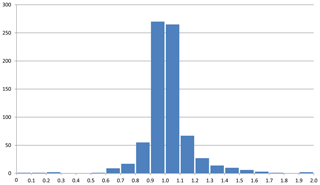Figure 5Frequency of occurrence of the factors for the non-zeolite entries listed in Table S1 and shown in Fig. 4. The three outliers with factors >2 are omitted.

6 Conclusions

The evaluation of 927 hydrous minerals and inorganic compounds showed that their H2O content can be calculated from their mean refractive indices if the anhydrous part of the chemical composition is known. Thus, single crystals with dimensions <100µm, usually used in X-ray diffraction analyses, can be used to determine the number of H2O molecules per formula unit if higher amounts of the sample are not available for thermal analyses. Based on the excellent overall agreement between the observed and calculated H2O contents of 157 zeolites and 770 other hydrates and the unusual deviations of paulingite, pollucite, tschörtnerite, liottite, and lotharmeyerite, we suggest that when strong disagreements occur, the refractive index, composition, and/or crystal structure should be more carefully investigated.

Code availability

The program POLARIO (Fischer et al., 2018) calculates the mean refractive index from the chemical composition and the molar volume. It also determines the H2O content of hydrous minerals from the anhydrous part of the chemical composition. The program can be downloaded free of charge from https://www.brass.uni-bremen.de/ (Fischer et al., 2018). Version 1.1 of the program was uploaded to the website on 23 October 2018.

Supplement

Author contributions

RDS and RXF designed the research and calculated individual and mean electronic polarizabilities of cations and anions. All authors compiled the data presented in Table 1 and Table S1. RXF and MB did the calculations, and all authors contributed to the text of the paper.

Competing interests

The authors declare that they have no conflict of interest.

Financial support

This research has been supported by the Deutsche Forschungsgemeinschaft (grant no. FI442/21-2).

The article processing charges for this open-access publication were covered by the University of Bremen.

Review statement

This paper was edited by Etienne Balan and reviewed by Anthony Kampf and Diego Gatta.

References

Alberti, A.: Sodium-rich dachiardite from Alpe di Siusi, Italy, Contrib. Mineral. Petrol., 49, 63–66, 1975.

Alberti, A. and Vezzalini, G.: The crystal structure of amicite, a zeolite, Acta Crystallogr. B, 35, 2866–2869, 1979.

Alberti, A., Hentschel, G., and Vezzalini, G.: Amicite, a new natural zeolite, Neues Jb. Miner. Monat., 481–488, 1979.

Alietti, A. and Passagli, E.: A new occurrence of ferrierite, Am. Mineral., 52, 1562–1563, 1967.

Anderson, O. L.: Optical properties of rock-forming minerals derived from atomic properties, Fortschr. Mineral., 52, 611–629, 1975.

Baerlocher, C. and McCusker, L. B.: Database of zeolite structures, available at: http://www.iza-structure.org/databases/, last access: August 2019.

Ballirano, P., Merlino, S., Bonaccorsi, E., and Maras, A.: The crystal structure of liottite, a six-layer member of the cancrite group, Can. Mineral., 34, 1021–1030, 1996.

Beger, R. M.: The crystal structure and chemical composition of pollucite, Z. Kristallogr., 129, 280–302, 1969.

Boggs, R. C., Howard, D. G., Smith, J. V., and Klein G. L.: Tschernichite, a new zeolite from Goble, Columbia County, Oregon, Am. Mineral., 78, 822–826, 1993.

Bonaccorsi, E. and Orlandi, P.: Marinellite, a new feldspathoid of the cancrinite-sodalite group, Eur. J. Mineral., 15, 1019–1027, https://doi.org/10.1127/0935-1221/2003/0015-1019, 2003.

Bonardi, M., Roberts, A. C., Sabina, A. P., and Chao, G. Y.: Sodium rich dachiardite from the Francon Quarry, Montreal Island, Quebec, Can. Mineral., 19, 285–289, 1981.

Cabella, R., Lucchetti, G., Palenzona, A., Quartieri, S., and Vezzalini, G.: First occurrence of a Ba-dominant brewsterite: structural features, Eur. J. Mineral., 5, 353–360, https://doi.org/10.1127/ejm/5/2/0353, 1993.

Cámara, F., Bellatreccia, F., Della Ventura, G., and Mottana, A.: Farneseite, a new mineral of the cancrinite – sodalite group with a 14-layer stacking sequence: occurrence and crystal structure, Eur. J. Mineral., 17, 839–846, https://doi.org/10.1127/0935-1221/2005/0017-0839, 2005.

Černý, P.: The Tanco pegmatite at Bernic Lake, Manitoba. VIII. Secondary minerals from the spodumene-rich zones, Can. Mineral., 11, 714–726, 1972.

Černý, P.: The present status of the analcime-pollucite series, Can. Mineral., 12, 334–341, 1974.

Černý, P. and Povondra, P.: A polycationic, strontian heulandite; comments on crystal chemistry and classification of heulandite and clinoptilolite, Neues Jb. Miner. Monat., 1969, 349–361, 1969.

Černý, P. and Simpson, F. M.: The Tanco pegmatite at Bernic Lake, Manitoba X. pollucite, Can. Mineral., 16, 325–333, 1978.

Chen, T. T. and Chao, G. Y.: Tetranatrolite from Mont St-Hilaire, Québec, Can. Mineral., 18, 77–84, 1980.

Chukanov, N. V., Pekov, I. V., Sejkora, J., Plášil, J., Belakovskiy, D. I., and Britvin S. N.: Ferrierite-NH4, $\left({\mathrm{NH}}_{\mathrm{4}},{\mathrm{Mg}}_{\mathrm{0.5}}{\right)}_{\mathrm{5}}\left({\mathrm{Al}}_{\mathrm{5}}{\mathrm{Si}}_{\mathrm{31}}{\mathrm{O}}_{\mathrm{72}}\right)\cdot \mathrm{22}{\mathrm{H}}_{\mathrm{2}}\mathrm{O}$, a new zeolite from Northern Bohemia, Czech Republic, Can. Mineral., 57, 81–90, https://doi.org/10.3749/canmin.1800057, 2019.

Coombs, D. S., Alberti, A., Armbruster, T., Artioli, G., Colella, C., Galli, E., Grice, J. D., Liebau, F., Mandarino, J. A., Minato, H., Nickel, E. H., Passaglia, E., Peacor, D. R., Quartieri, S., Rinaldi, R., Ross, M., Sheppard, R. A., Tillmanns, E., and Vezzalini, G.: Recommended nomenclature for zeolite minerals: Report of the subcommittee on zeolites of the international mineralogical association, commission on new minerals and mineral names, Eur. J. Mineral., 10, 1037–1081 https://doi.org/10.1127/ejm/10/5/1037, 1998.

Cortesogno, L., Lucchetti, G., and Penco, A. M.: Associoazioni a Zeoliti nel “Gruppo di Voltri”: Caratteristiche Mineralogiche e Significato Genetico, Soc. Italiana di Mineralogia e Petrologia – Rendiconti, 31, 673–710, 1975.

Deffeyes, K. S.: Erionite from cenozoic tuffaceous sediments, Central Nevada, Am. Mineral., 44, 501–509, 1959.

Dunn, P. J., Peacor, D. R., Newberry, N., and Ramik, R. A.: Goosecreekite, a new calcium aluminum silicate hydrate possibly related to brewsterite and epistilbite, Can. Mineral., 18, 323–327, 1980.

Eberlein, G. D., Erd, R. C., Weber, F., and Beatty, L. B.: New occurrence of yugawaralite from the Chena Hot Springs Area, Alaska, Am. Mineral., 56, 1699–1717, 1971.

Effenberger, H., Giester, G., Krause, W., and Bernhardt, H. J.: Tschörtnerite, a copper-bearing zeolite from the Bellberg volcano, Eifel, Germany, Am. Mineral., 83, 607–617, 1998.

Eggleton, R. A.: Gladstone-Dale constants for the major elements in silicates: Coordination number, polarizability, and the Lorentz-Lorentz relation, Can. Mineral., 29, 525–532, 1991.

Ercit, T. S. and van Velthuizen, J.: Gaultite, a new zeolite-like mineral species from Mont Saint-Hilaire, Quebec, and its crystal structure, Can. Mineral., 32, 855–863, 1994.

Fischer, R. X., Burianek, M., and Shannon, R. D.: POLARIO, a computer program for calculating refractive indices from chemical compositions, Am. Mineral., 103, 1345–1348, https://doi.org/10.2138/am-2018-6587, 2018.

Gagné, O., Hawthorne, F., Shannon, R. D., and Fischer, R. X.: Empirical electronic polarizabilities: deviations from the additivity rule. I. ${\mathrm{M}}^{\mathrm{2}+}{\mathrm{SO}}_{\mathrm{4}}\cdot n{\mathrm{H}}_{\mathrm{2}}\mathrm{O}$, blödite ${\mathrm{Na}}_{\mathrm{2}}{\mathrm{M}}^{\mathrm{2}+}\left({\mathrm{SO}}_{\mathrm{4}}{\right)}_{\mathrm{2}}\cdot \mathrm{4}{\mathrm{H}}_{\mathrm{2}}\mathrm{O}$ and kieserite-related minerals with sterically strained structures, Phys. Chem. Miner., 45, 303–310, https://doi.org/10.1007/s00269-017-0919-9, 2018.

Galli, E. and Gualtieri, A. F.: Direnzoite, [NaK6MgCa2(Al13Si47O120)⋅36H2O], a new zeolite from Massif Central (France): Description and crystal structure, Am. Mineral., 93, 95–102, https://doi.org/10.2138/am.2008.2666, 2008.

Galli, E. and Loschi Ghittoni, A. G.: The crystal chemistry of phillipsites, Am. Mineral., 57, 1125–1145, 1972.

Galli, E. and Passaglia, E.: Stellerite from Villanova Monteleone, Sardinia, Lithos, 6, 83–90, 1973.

Galli, E., Passaglia, E., Pongiluppi, D., and Rinaldi, R.: Mazzite, a new mineral, the natural counterpart of the synthetic Zeolite Ω, Contrib. Mineral. Petr., 45, 99–105, 1974.

Galli, E., Quartieri, S., Vezzalini, G., and Alberti, A.: Gottardiite, a new high-silica zeolite from Antarctica: the natural counterpart of synthetic NU-87, Eur. J. Mineral., 8, 687–693, https://doi.org/10.1127/ejm/8/4/0687, 1996.

Grice, J. D., Gault, R. A., and Ansell, H. G.: Edingdonite: The first two Canadian occurrences, Can. Mineral., 22, 253–258, 1984.

Grice, J. D, Kristiansen, R., Friis, H., Rowe, R., Poirier, G. G., Selbekk, R. S., Cooper, M. A., and Larsen A. O.: Ferrochiavennite, a new beryllium silicate zeolite from syenite pegmatites in the Larvik plutonic complex, Oslo Region, Southern Norway, Can. Mineral., 51, 285–296, https://doi.org/10.3749/canmin.51.2.285, 2013.

Gude, A. J. and Sheppard, R. A.: Silica-rich chabazite from the Barstow formation, San Bernardino County, Southern California, Am. Mineral., 51, 909–915, 1966.

Gude, A. J. and Sheppard, R. A.: Woolly erionite from the Reese river zeolite deposit, Lander County, Nevada, and its relationship to other erionites, Clay. Clay Miner., 29, 378–384, 1981.

Harada, K., Iwamoto, S., and Kihara, K.: Erionite, phillipsite and gonnardite in the amygdales of altered basalt from Mazé, Niigata Prefecture, Japan, Am. Mineral., 52, 1785–1794, 1967.

Hey, M. H. and Bannister F. A.: Studies on the zeolites. Part III. Natrolite and metanatrolite, Mineral. Mag., 23, 243–289, 1932a.

Hey, M. H. and Bannister, F. A.: Studies on zeolites. Part II. Thomsonite (including faroelite) and gonnardite, Mineral. Mag., 23, 51–125, 1932b.

Hey, M. H. and Bannister, F. A.: Studies on the zeolites. Part VI. Edingtonite, Mineral. Mag., 23, 483–494, 1934.

Hey, M. H. and Bannister, F. A.: Studies on the zeolites. Part IX. Scolecite and metascolecite, Mineral. Mag., 24, 227–253, 1936.

Howard, D. G., Tschernich, R. W., Smith, J. V., and Klein G. L.: Boggsite, a new high-silica zeolite from Goble, Columbia County, Oregon, Am. Mineral., 75, 1200–1204, 1990.

Hurlbut, C. S.: Bikitaite, LiAlSi2O6⋅H2O, a new mineral from Southern Rhodesia, Am. Mineral., 42, 792–797, 1957.

Jambor, J. L. and Grew, E. S.: New mineral names. Si-dominant cancrinite, Am. Mineral., 78, 235, 1993.

Kamb, W. B. and Oke, W. C.: Paulingite, a new zeolite, in association with erionite and filiform pyrite, Am. Mineral., 45, 79–91, 1960.

Khomyakov, A. P., Pobedimskaya, E. A., Nadezhina, T. N., Terenteva, L. E., and Rastsvetaeva, R. K.: Structural mineralogy of high-Si cancrinite, Moscow Univ. Geol. Bull., 46, 71–75, 1991a.

Khomyakov, A. P., Semenov, E. I., Pobedimskaya, E. A., Nadezhina, T. N., and Rastsvetaeva, R. K.: Cancrisilite Na7[Al5Si7O24]CO3⋅3H2O – a new mineral of the cancrinite group, Zapiski Vsesoyuznogo Mineralogicheskogo Obshchestva, 120, 80–84, 1991b.

Khomyakov, A. P., Cámara, F., and Sokolova, E.: Carbobystrite, Na8[Al6Si6O24](CO3)⋅4H2O, a new cancrinite-group mineral species from the Khibina Alkaline Massif, Kola Peninsula, Russia: Description and crystal structure, Can. Mineral., 48, 291–300, https://doi.org/10.3749/canmin.48.2.291, 2010.

Kiseleva, I., Navrotsky, A., Belitsky, I. A., and Fursenko, B. A.: Thermochemistry and phase equilibria in calcium zeolites, Am. Mineral., 81, 658–667, 1996a.

Kiseleva, I., Navrotsky, A., Belitsky, I. A., and Fursenko, B. A.: Thermochemistry of natural potassium sodium calcium leonhardite and its cation-exchanged forms, Am. Mineral., 81, 668–675, 1996b.

Larsen, A. O., Nordrum, F. S., Döbelin, N., Armbruster, T., Petersen, O. V., and Erambert, M.: Haulandite-Ba, a new zeolite species from Norway, Eur. J. Mineral., 17, 143–153, https://doi.org/10.1127/0935-1221/2005/0017-0143, 2005.

Leavens, P. B., Hurlbut, C. S., and Nelen, J. A.: Eucryptite and bikitaite from King's Mountain, North Carolina, Am. Mineral., 53, 1202–1207, 1968.

Lengauer, C. L., Giester, G., and Tillmanns, E.: Mineralogical characterization of paulingite from Vinarická Hora, Czech Republic, Mineral. Mag., 61, 591–606, 1997.

Lengauer, C. L., Kolitsch, U., and Tillmanns, E.: Flörkeite, K3Ca2Na[Al8Si8O32]⋅12H2O, a new phillipsite-type zeolite from the Bellerberg, East Eifel volcanic area, Germany, Eur. J. Mineral., 21, 901–913, https://doi.org/10.1127/0935-1221/2009/0021-1952, 2009.

Mandarino, J. A.: The Gladstone-Dale relationship. Part I: Derivation of new constants, Can. Mineral., 14, 498–502, 1976.

Mandarino, J. A.: The Gladstone-Dale relationship. Part IV. The compatibility concept and its application, Can. Mineral., 19, 441–450, 1981.

Medenbach, O., Maresch, W. V., Mirwald, P. W., and Schreyer, W.: Variation of refractive index of synthetic Mg-cordierite with H2O content, Am. Mineral., 65, 367–373, 1980.

Men'chikov, Y. P.: Perlialite ${\mathrm{K}}_{\mathrm{9}}\mathrm{Na}\left(\mathrm{Ca},\mathrm{Sr}\right){\mathrm{Al}}_{\mathrm{12}}{\mathrm{Si}}_{\mathrm{24}}{\mathrm{O}}_{\mathrm{72}}\cdot \mathrm{15}{\mathrm{H}}_{\mathrm{2}}\mathrm{O}$, a new potassian zeolite from the Khibina massif, Zapiski Vsesoyuznogo Mineralogicheskogo Obshchestva, 113, 607–612, 1984.

Men'shikov, Y. P., Denisov, A. P., Uspenskaya, Y. I., and Lipatova, E. A.: Lovdarite, a new hydrous alkali-beryllium silicate, Doklady Akad. Nauk. SSSR, 213, 130–133, 1973.

Merkle, A. B. and Slaughter, M.: Determination and refinement of the structure of heulandite, Am. Mineral., 53, 1120–1138, 1968.

Merlino, S. and Orlandi, P.: Franzinite, a new mineral phase from Pitigliano (Italy), Neues Jb. Miner. Monat., 163–167, 1977a.

Merlino, S. and Orlandi, P.: Liottite, a new mineral in the cancrinite-davyne group, Am. Mineral., 62, 321–326, 1977b.

Nawaz, R. and Malone, J. F.: Gobbinsite, a new zeolite mineral from Co. Antrim, N. Ireland, Mineral. Mag., 46, 365–369, 1982.

Passaglia, E.: Roggianite, a new silicate mineral, Clay Miner., 8, 107–111, 1969.

Passaglia, E.: The crystal chemistry of chabazites, Am. Mineral., 55, 1278–1301, 1970.

Passaglia, E. and Pongiluppi, D.: Sodian stellerite from Capo Pula, Sardegna, Lithos, 7, 69–73, 1974.

Passaglia, E. and Vezzalini, G.: Roggianite: revised chemical formula and zeolitic properties, Mineral. Mag., 52, 201–206, 1988.

Passaglia, E., Pongiluppi, D., and Rinaldi, R.: Merlinoite, a new mineral of the zeolite group, Neues Jb. Miner. Monat., 355–364, 1977.

Passaglia, E, Galli, E., and Rinaldi, R.: Levynes and erionites from Sardinia, Italy, Contrib. Mineral. Petr., 43, 253–259, 1974.

Peacor, D. R., Dunn, P. J., Simmons, W. B., Tillmanns, E., and Fischer, R. X.: Willhendersonite, a new zeolite isostructural with chabazite, Am. Mineral., 69, 186–189, 1984.

Pekov, I. V., Olysych, L. V., Zubkova, N. V., Chukanov, N. V., Van, K., V., and Pushcharovsky, D. Y.: Depmeierite ${\mathrm{Na}}_{\mathrm{8}}\left[{\mathrm{Al}}_{\mathrm{6}}{\mathrm{Si}}_{\mathrm{6}}{\mathrm{O}}_{\mathrm{24}}\right]\left({\mathrm{PO}}_{\mathrm{4}},{\mathrm{CO}}_{\mathrm{3}}{\right)}_{\mathrm{1}-\mathrm{x}}\cdot \mathrm{3}{\mathrm{H}}_{\mathrm{2}}\mathrm{O}\left(x<\mathrm{0.5}\right)$: a new cancrinite-group mineral species from the Lovozero alkaline pluton of the Kola Peninsula, Geol. Ore Deposits, 53, 604–613, 2011.

Petersen, O. V., Giester, G., Brandstätter, F., and Niedermayr, G.: Nabesite, Na2BeSi4O10⋅4H2O, a new mineral species from the Ilímaussaq alkaline complex, South Greenland, Can. Mineral., 40, 173–181, https://doi.org/10.2113/gscanmin.40.1.173, 2002.

Pongiluppi, D.: A new occurrence of yugawaralite at Osilo, Sardinia, Can. Mineral., 15, 113–114, 1977.

Raade, G., Åmli, R., Mladeck, M. H., Din, V. K., Larsen, A. O., and Åsheim, A.: Chiavennite from syenite pegmatites in the Oslo region, Norway, Am. Mineral., 68, 628–633, 1983.

Reay, A. and Coombs, D. S.: Ashtonite, a strontian mordenite, Mineral. Mag., 38, 383–385, 1971.

Robinson, G. W. and Grice, J. D.: The barium analog of brewsterite from Harrisville, New York, Can. Mineral., 31, 687–690, 1993.

Rouse, R. C., Peacor, D. R., Dunn, P. J., Campbell, T. J., Roberts, W. L., Wicks, F. J., and Newbury, D.: Pahasapaite, a beryllophosphate zeolite related to synthetic zeolite rho, from the Tip Top pegmatite of South Dakota, Neues Jb. Miner. Monat., 433–440, 1987.

Rouse, R. C., Dunn, P. J., Grice, J. D., Schlenker, J. L., and Higgins, J. B.: Montesommaite, $\left(\mathrm{K},\mathrm{Na}{\right)}_{\mathrm{9}}{\mathrm{AL}}_{\mathrm{9}}{\mathrm{SI}}_{\mathrm{23}}{\mathrm{O}}_{\mathrm{64}}\cdot \mathrm{10}{\mathrm{H}}_{\mathrm{2}}\mathrm{O}$, a new zeolite related to merlinoite and the gismondine group, Am. Mineral., 75, 1415–1420, 1990.

Rüdinger, B., Tillmanns, E., and Hentschel, G.: Bellbergite-a new mineral with the zeolite structure type EAB, Mineral. Petrol., 48, 147–152, 1993.

Sahama, T. G. and Lehtinen, M.: Harmotome from Korsnäs, Finland, Mineral. Mag., 36, 444–448, 1967.

Sarp, H., Deferne, J., Bizouard, H., and Liebich, B. W.: La parthéite, CaAl2Si2O8⋅2H2O, un nouveau silicate naturel d'aluminium et de calcium, Schweiz. Miner. Petrog., 59, 5–13, 1979.

Seki, Y. and Oki, Y.: Wairakite-analcime solid solutions from low-grade metamorphic rocks of the Tanzawa mountains, central Japan, Mineral. J., 6, 36–45, 1969.

Shannon, R. D. and Fischer, R. X.: Empirical electronic polarizabilities of ions for the prediction and interpretation of refractive indices: oxides and oxysalts, Am. Mineral., 101, 2288–2300, https://doi.org/10.2138/am-2016-5730, 2016.

Shannon, R. C., Lafuente, B., Shannon, R. D., Downs, R. T., and Fischer, R. X.: Refractive indices of minerals and synthetic compounds, Am. Mineral., 102, 1906–1914, https://doi.org/10.2138/am-2017-6144, 2017.

Sheppard, R. A. and Gude, A. J.: Chemical composition and physical properties of the related zeolites offretite and erionite, Am. Mineral., 54, 875–886, 1969.

Staples, L. W. and Gard, J. A.: The fibrous zeolite erionite; its occurrence, unit cell, and structure, Mineral. Mag., 32, 261–281, 1959.

Tiba, T. and Matsubara, S.: Levyne from Dözen (Oki Islands), Japan, Can. Mineral., 15, 536–539, 1977.

Tomita, K., Koiso, M., Yamamoto, M., and Oba, N.: Laumontite in an andesite from Kinzan, Satsuma-Cho, Kagoshima Prefecture, J. Japan. Assoc. Mineral. Petr. Econ. Geol., 74, 443–449, 1979.

Vezzalini, G. and Oberti, R.: The crystal chemistry of gismondines: the non-existence of K-rich gismondines, B. Minéral., 107, 805–812, 1984.

Walter, F.: Weinebeneite, CaBe3(PO4)2(OH)2⋅4H2O, a new mineral species: mineral data and crystal structure, Eur. J. Mineral., 4, 1275–1283, https://doi.org/10.1127/ejm/4/6/1275, 1992.

Wise, W. S.: New occurrence of faujasite in southeastern California, Am. Mineral., 67, 794–798, 1982.

Wise, W. S., Nokleberg, W. J., and Kokinos, M.: Clinoptilolite and ferrierite from Agoura, California, Am. Mineral., 54, 887–895, 1969.

Yajima, S. and Nakamura, T.: New occurrence of ferrierite, Mineral. J., 6, 343–364, 1971.

Yang, Y. W., Evans, S. H., Downs, R. T., and Yang, H.: Lotharmeyerite, Ca(Zn,Mn)2(AsO4)2-(H2O,OH)2, Acta Crystallogr., E68, i9–i10, https://doi.org/10.1107/S1600536811054286, 2012.